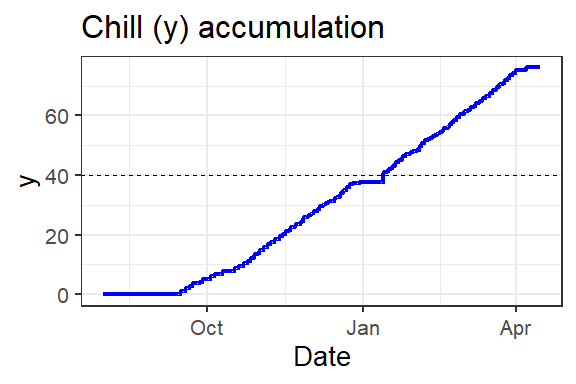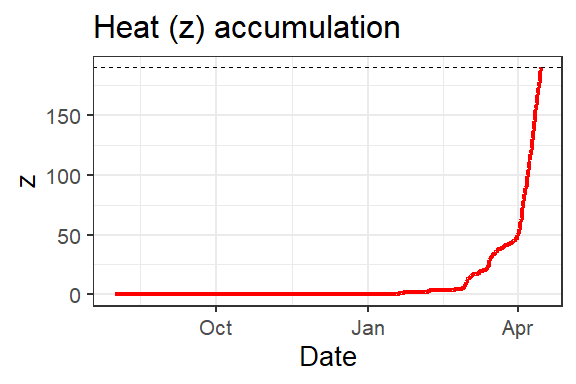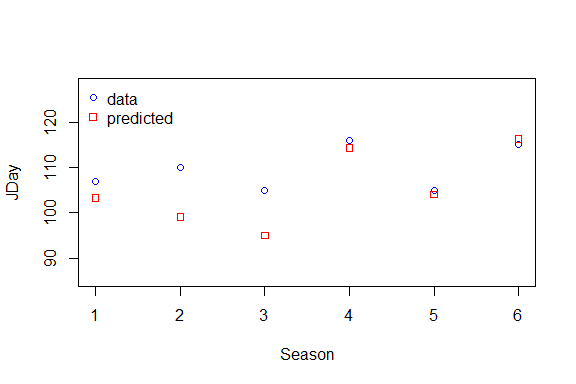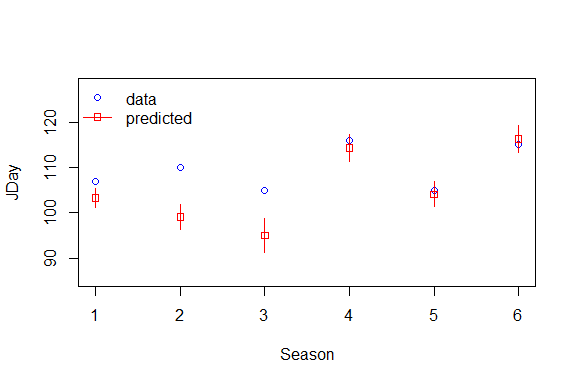# The PhenoFlex Model

The PhenoFlex Model constitutes a framework for modeling the spring phenology of deciduous trees, with a particular focus on fruit and nut trees. It uses the structure of the Dynamic Model for chill accumulation and the Growing Degree Hours model for heat accumulation. In order to relate spring phenology to chill and heat, and to account for varying theories about the relationship between these two agroclimatic phenomena, PhenoFlex includes a flexible transition function that defines responsiveness to heat (during ecodormancy) as a function of accumulated chill (during endodormancy). Unlike most previous applications of these models, PhenoFlex can flexibly fit the parameters of both models to observed spring phenology dates. The model can thus accommodate variation among the temperature responses of different species and cultivars.

The PhenoFlex Model is implemented in the chillR package, which also contains functions to fit the model parameters to observed spring phenology data. This vignette demonstrates the use of the PhenoFlex Model to predict spring phenology dates, as well as the procedure for fitting model parameters to data.

## Data preparation

We demonstrate the PhenoFlex functions using the example of cherry bloom data recorded at Campus Klein-Altendorf, the experimental station of the University of Bonn. This dataset, along with long-term records of daily minimum and maximum temperatures, is included in the chillR package (KA_bloom and KA_weather, respectively). Since the PhenoFlex model requires hourly temperatures, we use the stack_hourly_temps function from chillR to interpolate the daily data.

library(chillR)
library(ggplot2)
data(KA_weather)
data(KA_bloom)
hourtemps <- stack_hourly_temps(KA_weather, latitude=50.4)

In PhenoFlex, the chilling requirement is denoted $$y_c$$ and the heat requirement $$z_c$$. We first illustrate how the model can be used to predict spring phenology events, based on user-specified $$y_c$$ and $$z_c$$ values and using the usual parameters for the Dynamic Model and the Growing Degree Hours model (the default values in PhenoFlex). To run the analysis for one year only, we select all data for the 2009 season. The 2009 season is the dormancy season that ends in 2009. The number of months to consider for the season is specified in the genSeason function by the mrange parameter. Since the default (August to June) is appropriate here, this is not specified below.

yc <- 40
zc <- 190
iSeason <- genSeason(hourtemps, years=c(2009))
res <- PhenoFlex(temp=hourtemps$hourtemps$Temp[iSeason[]],
times=c(1: length(hourtemps$hourtemps$Temp[iSeason[]])),
zc=zc, stopatzc=TRUE, yc=yc, basic_output=FALSE)

The PhenoFlex function generates a list that tracks the accumulation of x (precursor to the dormancy-breaking factor), y (the dormancy-breaking factor; or Chill Portion), z (heat accumulation) and xs (the ratio of the formation to the destruction rate of x) over time. It also returns a bloomindex element, which points to the row in the temps input table that corresponds to estimated budbreak (or whatever the phenological stage of interest is).

DBreakDay <- res$bloomindex seasontemps<-hourtemps$hourtemps[iSeason[],]
seasontemps[,"x"]<-res$x seasontemps[,"y"]<-res$y
seasontemps[,"z"]<-res$z seasontemps<-add_date(seasontemps) ggplot(data=seasontemps[1:DBreakDay,],aes(x=Date,y=y)) + geom_line(col="blue",lwd=1.5) + theme_bw(base_size=20) + geom_hline(yintercept=yc,lty=2) + labs(title="Chill (y) accumulation")Chill accumulation over time. The dashed line respresents $$y_c$$, the critical amount of chill units for ecodormancy to be broken. ggplot(data=seasontemps[1:DBreakDay,],aes(x=Date,y=z)) + geom_line(col="red",lwd=1.5) + theme_bw(base_size=20) + geom_hline(yintercept=zc,lty=2) + labs(title="Heat (z) accumulation")Heat accumulation over time. The dashed line respresents the $$z_c$$, the critical amount of heat units for ecodormancy to be broken. # The phenologyFitter The PhenoFlex model can be fitted to phenological data, provided that sufficient observations are available for the cultivar of interest. For this kind of model, parameters are usually determined using an empirical solver. Solvers identify the best combination of model parameters by trying out different values and gradually adjusting these parameters, until the objective function - a measure of how far predictions are from the observed data - does not decrease further. In this framework, we fit the model using a generalized simulated annealing algorithm. In contrast to other algorithms, simulated annealing can deal with discrete data (we calculate the residual sum of squares between observed and predicted bloom days as objective function). Since simulated annealing is a stochastic solver, there is a risk of not finding the overall best parameter combination (global minimum of residual sum of squares). The generalisation of the basic solver reduces this risk. Still, the search should be repeated several times with different initial parameter values and random seeds. This iterative procedure requires lots of model runs, which can take substantial time and/or computing power. Here, we only demonstrate the functionality using a maximum of 10 iterations of the simulated annealing procedure. In order to achieve reliable parameters, we recommend at least 1000 iterations. We also recommend using many observations of a cultivar’s spring phenology (many more than in this example), and ideally to include winter seasons spanning a wide range of temperature conditions. For fitting the PhenoFlex model to phenological data, we have to generate a list of seasons as follows. We are only using 6 seasons in this example, but a real-life application should be based on at least 15 to 20 seasons or even more, comprising variable temperature profiles across years. SeasonList <- genSeasonList(hourtemps$hourtemps, years=c(2003:2008))

Parameters are then fitted using the generalized simulated annealing algorithm, which is called by the phenologyFitter function and requires several inputs:

• an integer vector of observed dates of bloom (or other phenological stages)
• a function describing the model to be fitted, which only takes as input a data.frame of dates and observed temperatures. Since the PhenoFlex model has more than one parameter, we have to wrap it into another function (PhenoFlex_GDHwrapper), which requires a temperature dataset x and a vector containing all the parameters of the PhenoFlex model. Within the wrapper function, this parameter vector is unpacked, with each value assigned to the respective parameter.
• a vector containing initial estimates for all parameters
• a vector containing lower bounds for all parameters
• a vector containing upper bounds for all parameters

The order, in which the PhenoFlex parameters have to be provided, is given in the description of the PhenoFlex_GDHwrapper function as follows:

1. yc - chilling requirement; critical value of y, which defines the end of chill accumulation - default value: 40
2. zc - heat requirement; critical value of z, which defines the end of heat accumulation - default value: 190
3. s1 - slope parameter that determines the transition from the chill accumulation to the heat accumulation period in PhenoFlex - default value: 0.5
4. Tu - optimal temperature of the Growing Degree Hours (GDH) model - default value: 25
5. E0 - time-independent activation energy of forming the PDBF - default value: 3372.8 (as in the widely used version of the Dynamic Model)
6. E1 - time-independent activation energy of destroying the PDBF - default value: 9900.3 (as in the widely used version of the Dynamic Model)
7. A0 - amplitude of the (hypothetical) process involved in forming the precursor to the dormancy-breaking factor in the Dynamic Model - default value: 6319.5 (as in the widely used version of the Dynamic Model)
8. A1 - amplitude of the (hypothetical) process involved in destroying the precursor to the dormancy-breaking factor (PDBF) in the Dynamic Model - default value: 5.939917e+13 (as in the widely used version of the Dynamic Model)
9. Tf - transition temperature parameter of the sigmoidal function in the Dynamic Model, also involved in converting PDBF to Chill Portions - default value: 4
10. Tc - upper threshold in the GDH model - default value: 36
11. Tb - base temperature of the GDH model - default value: 4
12. slope - slope parameter of the sigmoidal function in the Dynamic Model, which determines what fraction of the PDBF is converted to a Chill Portion - default value: 1.6

Note that the PhenoFlex_GDHwrapper can only fit the version of the PhenoFlex model that uses a heat model based on the GDH concept. For use of the Gaussian heat model that can also be considered by PhenoFlex, use the PhenoFlex_GAUSSwrapper function.

For this example, we initiate the procedure with the default values, and we set upper and lower bounds as follows:

par <-   c(40, 190, 0.5, 25, 3372.8,  9900.3, 6319.5, 5.939917e13,  4, 36,  4,  1.60)
upper <- c(41, 200, 1.0, 30, 4000.0, 10000.0, 7000.0,       6.e13, 10, 40, 10, 50.00)
lower <- c(38, 180, 0.1, 0 , 3000.0,  9000.0, 6000.0,       5.e13,  0,  0,  0,  0.05)

Now, we can run the fitter:

Fit_res <- phenologyFitter(par.guess=par,
modelfn = PhenoFlex_GDHwrapper,
bloomJDays=KA_bloom$pheno[which(KA_bloom$Year %in% c(2003:2008))],
SeasonList=SeasonList, lower=lower, upper=upper,
control=list(smooth=FALSE, verbose=FALSE, maxit=10,
nb.stop.improvement=5))
## Loading required namespace: parallel

Note that the list control regulates the behavior of the GenSA algorithm, the solving engine we are using here. Note further that smooth=FALSE must be set for the PhenoFlex model, since the objective function, the residual sum of squares between predicted and observed bloom days is calculated based on discrete data (days) and is thus not smooth. verbose controls whether messages from the algorithm are shown. maxit defines the maximum number of iterations of the algorithm and should have a value of at least 1000. nb.stop.improvement is the number of search steps of the algorithm, after which the solver is stopped when there is no further improvement of the fit.

In the example above, the values are chosen in a way that allows the fitter to finish quickly. Thus, the result may not be particularly meaningful. More reasonable parameters are, for instance, the default values of phenologyFitter:

control=list(smooth=FALSE, verbose=TRUE, maxit=1000,
nb.stop.improvement=250)

phenologyFitter can also be used with other models. For instance, in order to fit the StepChill model, we could add

modelfn=StepChill_Wrapper

to the argument list of phenologyFitter and adapt the parameter vectors par, upper and lower accordingly.

The result of a fitting procedure can be summarized as follows

summary(Fit_res)

Phenology Fitter

RMSE     :  6.277901
R^2      :  3.875698

data versus predicted:
data predicted      delta
1  107 103.29167  3.7083333
2  110  99.12500 10.8750000
3  105  95.04167  9.9583333
4  116 114.33333  1.6666667
5  105 104.08333  0.9166667
6  115 116.29167 -1.2916667

with data being the days where bloom or any other phenological event was observed, predicted the day that was predicted by the model and delta the difference between data and predicted, i.e. the residual error. These results can also be visualized using the plot function

plot(Fit_res)# Computing Errors

Model prediction are usually uncertain, and the possible errors this may produce should be expressed. To estimate these errors, we use a bootstrapping technique, which involves the following steps:

1. we first predict bloom dates using our fitted PhenoFlex framework (see above)
2. for all years in our calibration dataset, we calculate the residuals of the predictions (observed minus predicted bloom dates)
3. we then take the whole population of these residuals and draw as many random samples from it as we have years in our calibration dataset. In drawing these samples, it is important that they are drawn with replacement, i.e. all values that were calculated in step 2 are available each time a sample is drawn.
4. we add the residuals that we obtained in step 3 to the phenological dates in our calibration dataset
5. we use the resulting dates to again fit the parameters of the PhenoFlex framework, make predictions for the validation dataset and record the results for each year
6. we repeat steps 3-5 many times, recording prediction results for each repetition
7. based on the population of predicted bloom dates for each year, we calculate standard deviations of the resulting distribution, as well as the $$16^{th}$$ and $$84^{th}$$ percentiles, which provide estimates of the standard error. More details on this procedure and the whole PhenoFlex concept will eventually be provided in a peer-reviewed paper

In short, the residuals are bootstrapped and then the fit is repeated. This procedure is performed boot.R times.

Fit_res.boot <- bootstrap.phenologyFit(Fit_res, boot.R=10,
control=list(smooth=FALSE, verbose=TRUE, maxit=10,
nb.stop.improvement=5),
lower=lower, upper=upper, seed=1726354)
## ================================================================================

Like for the result of phenologyFitter there is also a summary and a plot function for bootstrap.phenologyFit.

summary(Fit_res.boot)
            par          Err          q16          q84
1  3.911677e+01 9.436347e-01 3.823354e+01 4.019700e+01
2  1.955518e+02 7.751765e+00 1.811036e+02 1.971122e+02
3  3.217589e-01 3.059922e-01 1.165326e-01 6.555805e-01
4  2.500000e+01 3.385816e+00 2.285960e+01 2.769650e+01
5  3.372800e+03 1.019385e+02 3.314583e+03 3.372800e+03
6  9.900300e+03 1.438488e+02 9.882464e+03 9.900300e+03
7  6.278621e+03 2.503686e+02 6.201676e+03 6.576603e+03
8  5.939917e+13 1.314934e+08 5.939912e+13 5.939930e+13
9  4.000000e+00 2.145572e+00 3.746031e+00 7.675494e+00
10 3.600000e+01 3.328906e+00 3.131869e+01 3.717341e+01
11 4.000000e+00 1.861776e+00 3.101301e+00 6.164235e+00
12 1.600000e+00 1.916526e+01 1.993704e+00 4.441563e+01

with par being the estimated parameter values, Err the standard deviation of the bootstrap distribution and q16 and q84 the 16. and 84. percentiles, respectively.

plot(Fit_res.boot)This plot now shows observed bloom dates, as well as bloom dates predicted with the PhenoFlex model, including an estimate of prediction uncertainty (shown as error bars).